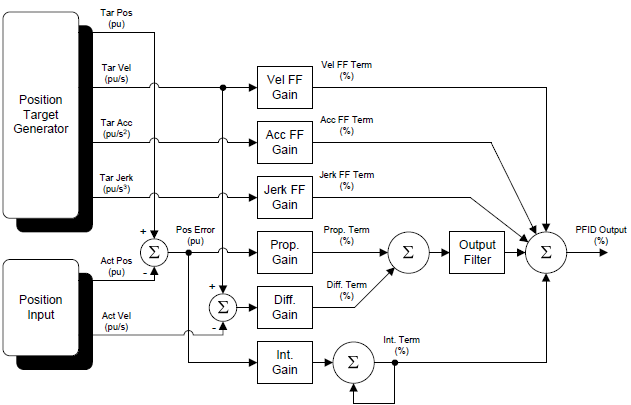# PID Algorithm

As i am doing my last sem project (B.E) on RMC70, can i get the Algorithm for PID controller used in RMC70 Series so that i can include that information in my project report which will be a plus mark for me.

Here is a diagram of the basic algorithm. The final PFID output range of +/- 100 % is converted to +/-10 V.
Is this sufficient for your needs?Can i have a brief explain of this circuit so that i can include your explanation in my report.

Each millisecond (called the loop time, which can actually be set to 0.25, 0.5, 1, 2, or 4 milliseconds depending on the RMC), the controller uses the Proportional Gain, Integral Gain, Differential Gain (more commonly known as derivative gain), Velocity Feed Forward, and Acceleration Feed Forward to calculate values (terms) that are all summed together to create the Control Output.

Each individual gain or feed forward is applied as follows:

• Proportional Gain:
The Proportional Gain is multiplied by the position error, which is the Target Position minus the Actual Position. Therefore, the greater the position error, the more Control Output will be generated to try to get the Actual Position to the Target Position.
• Integral Gain:
The Integral Gain is the integrated position error. Therefore, the longer a position error exists, the more Control Output will be generated to try to get the Actual Position to the Target Position.
• Differential Gain:
The Differential Gain is multiplied by the velocity error, which is the Target Velocity minus the Actual Velocity.
• Velocity Feed Forward
The Velocity Feed Forward is multiplied by the Target Velocity. Notice that the Velocity Feed Forward has nothing to do with the feedback. The Velocity Feed Forward works especially well on hydraulic cylinders (and motors in velocity mode) because the Actual Velocity is roughly proportional to the Control Output. Therefore, it is possible to know ahead of time approximately how much Control Output will be needed, since the Target Velocity is already known. This is what the Feed Forward does.
• Acceleration Feed Forward
Just like Velocity Feed Forward, but applied to the acceleration, using the Target Acceleration.
• Jerk Feed Forward
Just like Velocity Feed Forward, but applied to the jerk, using the Target Jerk (first derivative of acceleration). The Jerk Feed Forward is usually not used.

The Actual Velocity is calculated from the position, which results in quantization error. This error is multiplied by the Differential Gain, which results in noise in the Control Output. The Output Filter can be used to filter the Differential Gain (and Proportional), which reduces the noise and allows the Differential Gain to be increased more.

A PID-only system will always have a position error when the position is changing. The Feed Forwards make it possible for the Actual to track the Target without a lag.

Why only the velocity error is provided to the differential gain in PFID Algorithm?
What happens if the position error is provided to the differential gain

Then it wouldn’t be a differential gain. The term “differential” or “derivative” means the first derivative of the feedback quantity, which is normally position. The first derivative of position is velocity.